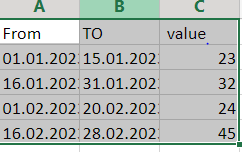# Comparing dates from 2 columns

HI,
I have an excel with 2 columns from and to and there is a 3rd column with valuesi have to check in between which date todays date come and get that corresponding value from clm 3 “value” eg:if today date is 14.02 then the date falls between 01.02 and 20.02 column so i need value 24. Is there any method to do this

Yes, there is a method to do this in UiPath. Here are the steps:

1. Use “Read Range” activity to read the Excel file into a DataTable.
2. Loop through the DataTable rows using “For Each Row” activity.
3. Inside the loop, use “DateTime.ParseExact” method to convert the “From” and “To” dates in each row to DateTime format.
4. Use “DateTime.Today” property to get today’s date and compare it with the “From” and “To” dates in the current row to check if it falls within the range.
5. If it falls within the range, get the corresponding value from the “Value” column in the current row and store it in a variable.
6. Once the loop is finished, the variable will contain the corresponding value for today’s date.

Here is some sample code in VB.Net,:

``````Dim dt As DataTable = excelDT //replace with your DataTable variable
Dim value As Integer

For Each row As DataRow In dt.Rows
Dim from As DateTime = DateTime.ParseExact(row("From"), "dd.MM.yyyy", Nothing)
Dim [to] As DateTime = DateTime.ParseExact(row("To"), "dd.MM.yyyy", Nothing)
If DateTime.Today >= from AndAlso DateTime.Today <= [to] Then
value = row("Value")
Exit For //exit the loop once a match is found
End If
Next

//value variable will contain the corresponding value for todays date

``````

Happy Automation, Cheers!

@Amruta_George1

3. inside use if condition with `CDate(CurrentRow("From").ToString)<Now and Now>CDate(CurrentRow("From").ToString)`(Eg:`CDate("2/13/2023")<Now and Now>CDate("2/15/2023")`)
4. In then side you can get `CurrentRow("value").ToString` using assign and then use break to stop